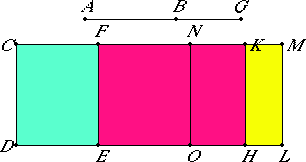# Proposition 102

The square on the straight line which produces with a medial area a medial whole, if applied to a rational straight line, produces as breadth a sixth apotome.

Let AB be the straight line which produces with a medial area a medial whole, and CD a rational straight line, and to CD let CE be applied equal to the square on AB and producing CF as breadth.

I say that CF is a sixth apotome.

X.78

Let BG be the annex to AB. Then AG and GB are straight lines incommensurable in square which make the sum of the squares on them medial, twice the rectangle AG by GB medial, and the sum of the squares on AG and GB incommensurable with twice the rectangle AG by GB.Now to CD apply CH equal to the square on AG and producing CK as breadth, and KL equal to the square on BG. Then the whole CL equals the sum of the squares on AG and GB. Therefore CL is also medial.

X.22

And it is applied to the rational straight line CD producing CM as breadth, therefore CM is rational and incommensurable in length with CD.

II.7

Since CL equals the sum of the squares on AG and GB, and, in these, CE equals the square on AB, therefore the remainder FL equals twice the rectangle AG by GB. And twice the rectangle AG by GB is medial, therefore FL is also medial.

X.22

And it is applied to the rational straight line FE producing FM as breadth, therefore FM is rational and incommensurable in length with CD.

Since the sum of the squares on AG and GB is incommensurable with twice the rectangle AG by GB, CL equals the sum of the squares on AG and GB, and FL equals twice the rectangle AG by GB, therefore CL is incommensurable with FL.

But CL is to FL as CM is to MF, therefore CM is incommensurable in length with MF. And both are rational.

X.73

Therefore CM and MF are rational straight lines commensurable in square only, therefore CF is an apotome.

I say next that it is also a sixth apotome.

Since FL equals twice the rectangle AG by GB, bisect FM at N, and draw NO through N parallel to CD, therefore each of the rectangles FO and NL equals the rectangle AG by GB.

And, since AG and GB are incommensurable in square, therefore the square on AG is incommensurable with the square on GB.

But CH equals the square on AG, and KL equals the square on GB, therefore CH is incommensurable with KL.

But CH is to KL as CK is to KM, therefore CK is incommensurable with KM.

Since the rectangle AG by GB is a mean proportional between the squares on AG and GB, CH equals the square on AG, KL equals the square on GB, and NL equals the rectangle AG by GB, therefore NL is also a mean proportional between CH and KL. Therefore CH is to NL as NL is to KL.

X.18

And for the same reason as before the square on CM is greater than the square on MF by the square on a straight line incommensurable with CM.

X.Def.III.6

And neither of them is commensurable with the rational straight line CD set out, therefore CF is a sixth apotome.

Therefore, the square on the straight line which produces with a medial area a medial whole, if applied to a rational straight line, produces as breadth a sixth apotome.

Q.E.D.

## Guide

This proposition is used in X.111.